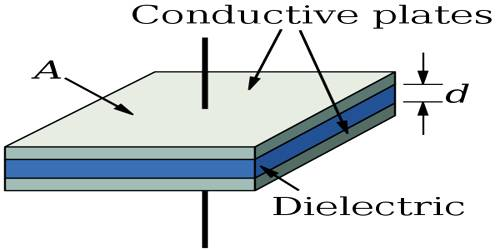# Significance of Dielectric Constant

Significance of dielectric constant

Any non-conducting or insulating medium between any two point charges keeps, the charges isolated. This type of medium is called dielectric medium. The dielectric must be a good electric insulator so as to minimize any DC leakage current through a capacitor. Examples of dielectric materials are glass, ebonite, rubber, oil, wax etc. Dielectric materials are also used in the construction of radio-frequency transmission lines.

Dielectric constant does not depend on the unit of measurement. Presence of dielectric medium between two charges decreases the force between them by ε, times than that in air or vacuum. On the other hand, the presence of dielectric medium between the plates of a capacitor increases the capacitance by εr times than that in air or vacuum.

“The dielectric constant of a medium is 2.5” – this statement means that the force between two charges at a particular distance in that medium is 2.5 times smaller than that in air or vacuum. That means the ratio of the force between two charges placed at an equal distance in the air or in the vacuum and in any other medium is 2.5 times.

In terms of capacitance, this statement means that capacitance of a parallel plate capacitor with this dielectric between the plates is 2.5 times more than that with air or vacuum between the plates of the capacitor. That means the ratio of capacitances of a capacitor filled with that medium and with air or vacuum is 2.5 time.